# Structure in C

## Structure in C with Examples

In this article, I am going to discuss Structure in C Program with Examples. Please read our previous article where we discussed Error Handling in C Program. At the end of this article, you will understand the following pointers in detail.

1. What is Structure in C?
2. Real-time Example to understand Structure
3. How memory is created for a structure in C?
4. How to declare a variable of type structure?
5. Where the structure variable is created inside the Main memory?
6. How to access the member of a structure?
7. What is the operator used for accessing the member?
8. Self-Referential Structure in C
9. Nested Structure in C
10. Structure and Pointer
##### What is Structure in C?

The structure can be defined as a collection of related data members under one name. Those data members may be of similar type or may be of dissimilar type. So usually it is defined as a collection of dissimilar data items under one name.

Structure in C is used for defining user-defined data types. Apart from the primitive data type we have in any programming language, for example in C language, we have primitive data types such as integer, float, double, etc. Using these primitive data types, we can also define our own data type depending on our own requirements. And this is possible in C because of Structure.

In this course, I am going to use Structure more frequently. In this article, we will learn how to define the structure and what does it mean by the size of a structure like how much memory it consumes? Then we will discuss how to declare a structure and how to access the members of a structure?

##### Example to understand Structure:

Let us take an example of a rectangle. A rectangular is a figure having two properties i.e. length and breadth as shown in the below image.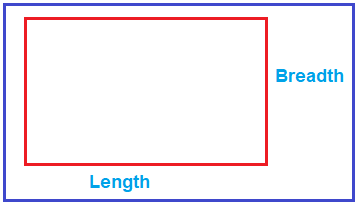So, a rectangle will have length and breadth, which means these two things (length and breadth) together defines a rectangle. So, in your programs, if you need something like a rectangle then you need to define a structure for it because a rectangle is not defined by just one value rather than is defined by a set of related values i.e. length and breadth. So, you can group them together under one name and define it as a structure.

For example, you need to define a structure for a rectangle as shown below.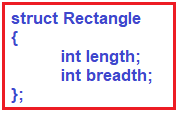As shown in the above image, we are creating a struct Rectangle, inside this, we have integer type length, an integer type breadth. Now, this struct rectangle is having two members (length and breadth).

We are taking length and breadth as an integer type, but you can also take them as float or double or any other type depending on your business requirement. We are assuming that these are simple values that are integral types. So, a structure rectangle is defined by its length and breadth or we can say these are two data members together define a rectangle. This is the definition of a structure

##### How much memory this rectangle will be consuming?

It is having two integer members. Integer takes 2 bytes or it may take 4 bytes depending upon the Operating system. But let’s assume it takes 2 bytes. So, it will take a total of 4 bytes (2 bytes for length plus 2 bytes for breadth) of memory.

So, this rectangle structure is taking 4 bytes of memory. Right now, it is not consuming any memory because it is just a definition. So, if we create a variable if this type, then it will be occupying that much of memory. We have learned how to know its size.

Note: The size of a structure is the total amount of memory consumed by all its member.

##### How to declare a variable of type structure?

Please have a look at the below code.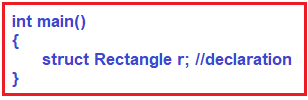As you can see in the above code, inside the main() method we declare a variable. So, the method of structure variable declaration is writing struct as a keyword then gives the structure name i.e. Rectangle, and followed by the variable name i.e. in this case ‘r’. This is the declaration of a structure variable. Now, this r will occupy the memory space and this will be created in the memory.

We can also declare and initialize at the same time as shown below.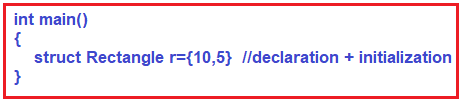As you can see in the above image, the struct variable ‘r’ is created with the values 10 and 5. Here the value 10 is assigned to length and the value 5 is assigned to breadth is 5.

##### Where this r variable is created inside the Main memory?

The variable r is going to be created inside the stack frame of the main memory as shown in the below image.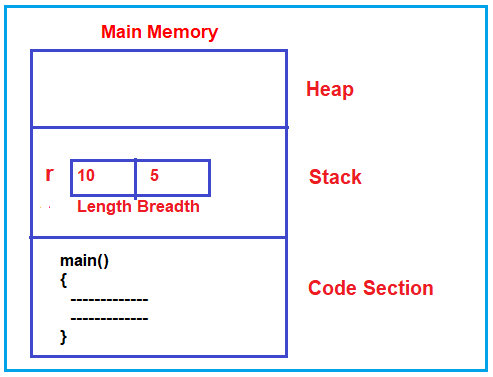##### How to access the member of the structure?

Suppose, you want to access the length of the structure i.e. you want to modify the length value to 15. For accessing the member of a structure, we need to use the structure variable name and the dot operator followed by the structure member name. The following code shows how to modify the length of the structure.

r.length=5

##### What is the operator used for accessing the member?

The dot (.) operator is used for accessing a member of a structure. So, if you want to read and write the members of a structure then you need to use the dot operator. Let us write the complete example which will calculate the area of a rectangle.

```#include <stdio.h>
struct Rectangle
{
int length;
};

int main()
{
struct Rectangle r = { 10, 5 };
r.length = 20;
printf ("Area of Rectangle: %d", r.length * r.breadth);
return 0;
}
```

Output: Area of Rectangle: 200

I hope you understand the basics of structure. Now let us proceed and understand the structure in-depth with more examples.

##### Structure in C:

In the C programming language, we are having three types of datatypes:

1. Primitive Datatypes
2. Derived Datatypes
3. User-Defined Datatypes

The structure is a collection of dissimilar elements in contiguous memory locations, under a single name. They are user-defined data types. The name of the structure (or the tag name) is treated as a data type, and the elements of the structure are known as its members. No memory is allocated during the definition of the structure. Memory is only allocated when its variables are created (which is usually preceded by the keyword struct). The variables of the structure types occupy the memory size which is equal to the sum of the size of all its members. At one time simultaneously data can be stored in all its members. The members can be accessed by using the dot (.) operator.

All primitive data types are used to manipulate basic data types i.e. char, int, float. All derived datatypes work for primitive datatypes. In the real world, every piece of information will be there in the form of objects. Every object has its own properties and behavior. No, any primitive or derived datatypes support real-time object information. When the primitive or derived datatypes are not supporting user requirements then go for user-defined datatypes.

• A Structure is a collection of different types of data elements in a single entity.
• A Structure is a combination of primitive and derived datatype variables.
• By using structures, we can create user-defined data types.
• The size of the structure is the sum of all member variable sizes.
• The least size of the structure is 1byte.
• In the ‘C’ programming language, it is not possible to create an empty structure.
• C language structure contains data members only but in C++, data members, and member functions.

For example, You want to store the information about a person about his/her name, citizenship number, and salary. You can create this information separately but, a better approach will be a collection of this information under a single name because all this information is related to the person.

##### Structure Definition in C

Keyword struct is used for creating a structure.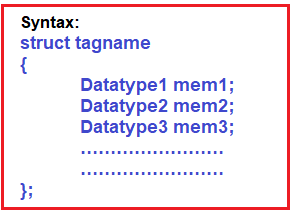According to the syntax of the structure, a semicolon must be required at the end of the structure body.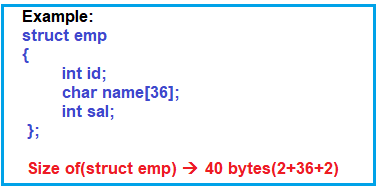##### Another Example: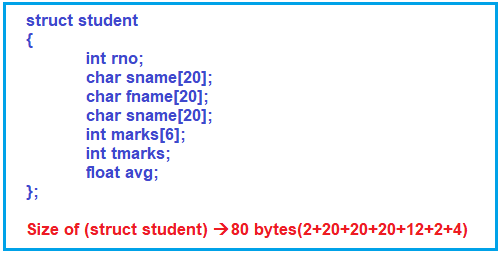##### Syntax to create structure variable

When a structure is defined, it creates a user-defined type but, no storage is allocated.

Syntax: struct tagname variable;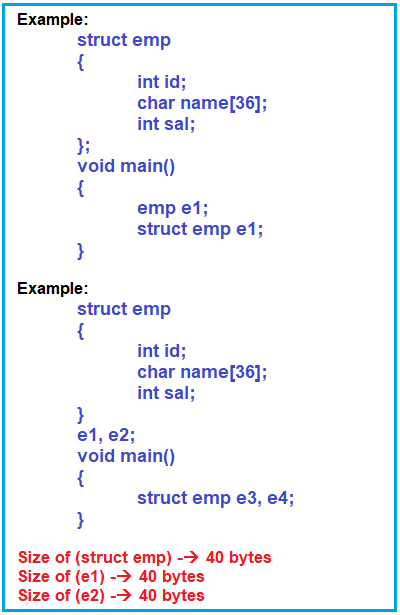When we are creating the structure variable at the end of the structure body, then it becomes a global variable i.e., e1, e2. When we are creating the structure variable within the body of the function, then it is an auto variable that is local to a specific function i.e. e3, e4.

##### Syntax to create structure type pointer

Syntax: struct tagname *ptr;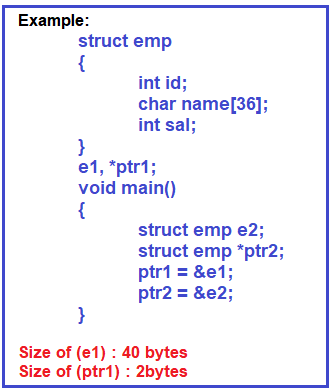Note: The size of the user-defined pointer is 2 bytes only because it holds an address.

##### Syntax to create structure type array

Syntax: struct tagname arr[size];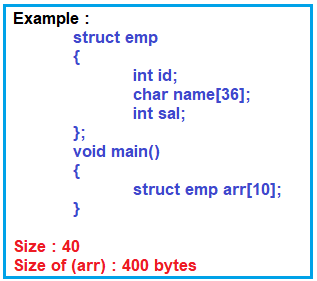##### Creating the Array Dynamically
```struct emp
{
int id;
char name;
int sal;
};
void main()
{
struct emp* arr;
arr = (struct emp*) calloc(10, sizeof(struct emp));
free(arr);
}
```
##### Syntax to initialize a structure variable

Syntax: struct tagname variable = {value1, value2, value3…..};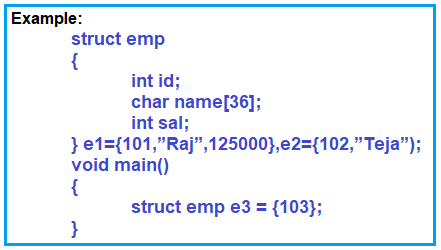In the initialization of the structure variable, if a specific number of members are not initialized, then remaining all members are initialized with 0 or null. If the value type member is not initialized, then it becomes 0, if string type data is not initialized, then it becomes null.

##### Syntax to access structure members

There are two types of operators used for accessing members of a structure. By using the following operators, we can access structure members:

1. Struct to the member(.)
2. Pointer to the member(->)

If the variable is normal operator struct to member operator whereas If the variable is a pointer type, then go for the pointer to member operator.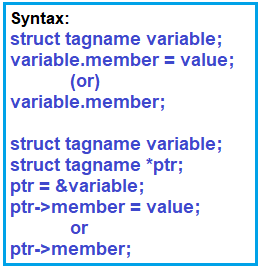##### Example:
```#include <stdio.h>
struct emp
{
int id;
char name;
int sal;
}
e1 ={101, "Raj", 12500};
e2 ={102, "Teja"};
void main ()
{
struct emp e3, e4, e5, e6;
e3.id = 103;
e3.name = Rajesh;		//Error
e3.name = "Rajesh";
strcpy (e3.name, "Rajesh");
e3.sal = 14000;
e4 = e3 + 1;		//error
e4 = e3.id + 1;		//Error
e4.id = e3.id + 1;		//yes
e4.name = e3.name;		//Error
strcpy (e4.name, e3.name);
e4.sal = e1 + e2;		//Error
e4.sal = e1.sal + e2.sal;	//yes
e5 = e4;

//We can assign one structure variable to another structure variable of same type.e4 == e5;	//Error
e4.id = e5.id;		//yes
e3.name > e4.name;		//error
strcmp (e3.name, e4.name);
e3.sal < e2.sal;		//yes
}
```

Any kind of manipulations can be performed on structure members. Except for the assignment, no other operations can be performed on structure variables. When two variables are the same structure type then it is possible to assign one variable data to another variable.

##### Example:
```#include <stdio.h>
struct emp
{
int id;
char name;
int sal;
};
void main ()
{
struct emp e1;
struct emp *ptr;
ptr = &e1;
//e1.id = 101;
ptr->id = 101;
strcpy (ptr->name, "Rajesh");
//strcpy(e1.name,"Rajesh");
//e1.sal=12500;
ptr->sal = 12500;
}
```
##### typedef

It is a keyword. By using this keyword, we can create a user-defined name for the existing data type. Generally, the typedef keyword is used to create an alias name for the existing datatype. The programmer generally use typedef while using structure in C language.

Syntax: typedef datatype user_defined_name;

##### Example:
```#include<stdio.h>
#include<conio.h>
typedef int myint;
int main ()
{
int x;
myint y;
typedef myint smallint;
smallint z;

printf ("Enter 2 values:");
scanf ("%d%d", &x, &y);
z = x + y;
printf ("sum value is : %d", z);
return 0;
}
```
###### Output: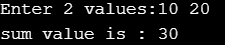##### Example:
```#include<stdio.h>
#define MYCHAR char
typedef char byte;
int main ()
{
char ch1 = 'A';
MYCHAR ch2 = 'b';
byte ch3;
ch3 = ch2 - ch1 + 20;
printf ("char1:%c char2:%c char3:%c", ch1, ch2, ch3);
return 0;
}
```

Output: char1:A char2:b char3:5

By using #define, we can’t create an alias name because, at the time of preprocessing, the identifier is replaced with the replacement text. The #define is under the control of the preprocessor, typedef is under the control of the compiler.

##### Note:
• When we are working with structures mentioning the tagname is optional, if tagname is not given, then the compiler creates a nameless structure.
• When we are working with nameless structures, it is not possible to create a structure variable whether the body of the function, i.e. global variables are possible to create.
• When the structure body is started with a typedef keyword, then it is not possible to create a structure variable at the end of the body i.e. global variable is not possible to create.
• In the ‘C’ programming language it is not possible to create an empty structure because the least size of the structure is 1 byte.
• For the creation of structure, it doesn’t occupy any physical memory.
• When we are working with the structure, physical memory will occupy.
• When we are creating variables but for initialization of members, we required physical memory.
##### Self-Referential Structure in C

Placing two structure type pointer has a member of the same structure is called a self-referential structure. By using left referential structure, we can handle any type of data structure.

##### Example:
```struct emp
{
int id;
char name;
int sal;
struct emp *ptr;
};
//Size of (struct emp) : 42 bytes

struct emp
{
int id;
char name;
int sal;
};

void abc ()
{
struct emp e1, e2;
}

void main ()
{
struct emp e1, e2;
}
```

Note: When we are creating the structure in the global scope, then it is possible to access the program in any function.

```void main ()
{
struct emp
{
int id;
char name;
int sal;
};
struct emp e1, e2;
}

void abc ()
{
struct emp e1, e2;
}
```

When the structure is created within the body of the function, then that structure needs to be accessed in the same function only.

##### Program:
```#include<stdio.h>
typedef struct
{
int id;
char name;
int sal;

} EMP;
EMP getdata ()
{
EMP te;
printf ("Enter EMP ID:");
scanf ("%d", &te.id);
printf ("Enter EMP name:");
scanf ("%s", te.name);
printf ("Enter EMP salary:");
scanf ("%d", &te.sal);
return te;
}

void showdata (EMP te)
{
printf ("\nID : %d NAME:%s SALARY:%d", te.id, te.name, te.sal);
}

int sumsal (int s1, int s2)
{
return (s1 + s2);
}

int main ()
{
EMP e1, e2;
int tsal;
e1 = getdata ();
e2 = getdata ();
showdata (e1);
showdata (e2);
tsal = sumsal (e1.sal, e2.sal);
printf ("\nSum Salary + %d", tsal);
}
```
###### Output: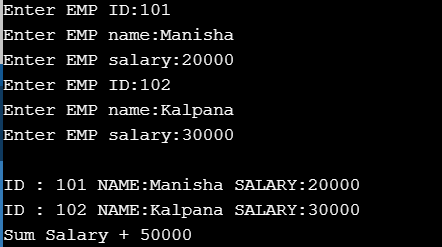##### Nested Structure in C

It is a procedure of placing a structure within an existing structure body. When we are working with a nested structure, the size of the structure is the sum of inner structure properties and outer structure properties are to be calculated. When we are working with a nested structure, it is not possible to access inner structure members directly by using the outer structure variable. In order to access inner structure members by using the outer structure variable, we are required to create an inner structure variable within the body only. The inner structure variable does not have to access outer structure members directly or indirectly. Structures can be nested within other structures in C programming.

##### Example:
```#include <stdio.h>
#include <string.h>
struct Employee
{
int id;
char name;
struct Date
{
int dd;
int mm;
int yyyy;
} doj;
} e1;

int main ()
{
//storing employee information
e1.id = 101;
strcpy (e1.name, "Krish");	//copying string into char array
e1.doj.dd = 10;
e1.doj.mm = 11;
e1.doj.yyyy = 2014;

//printing first employee information
printf ("employee id : %d\n", e1.id);
printf ("employee name : %s\n", e1.name);
printf ("employee date of joining (dd/mm/yyyy) : %d/%d/%d\n", e1.doj.dd, e1.doj.mm, e1.doj.yyyy);
return 0;
}
```
###### Output: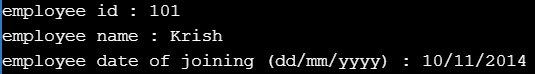##### Structure and Pointer

Pointers can be accessed along with structures. Structure’s member through a pointer can be used in two ways:

1. Referencing pointer to another address to access memory.
2. Using dynamic memory allocation.
##### Example:
```#include <stdio.h>
#include <string.h>
struct student
{
int id;
char name;
float percentage;
};

int main ()
{
int i;
struct student record1 = { 1, "Raju", 90.5 };
struct student *ptr;

ptr = &record1;

printf ("Records of STUDENT1: \n");
printf ("Id is: %d \n", ptr->id);
printf ("Name is: %s \n", ptr->name);
printf ("Percentage is: %f \n\n", ptr->percentage);

return 0;
}
```

Output: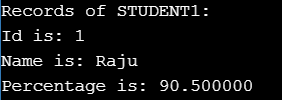Structure pointer member can also be accessed using -> operator.

(*ptr).a is same as ptr->a

(*ptr).b is same as ptr->b

In the next article, I am going to discuss Union in C language. Here, in this article, I try to explain Structure in C with Examples. I hope you enjoy this Structure in C with Examples article. I would like to have your feedback. Please post your feedback, question, or comments about this Structure in C with Examples article.